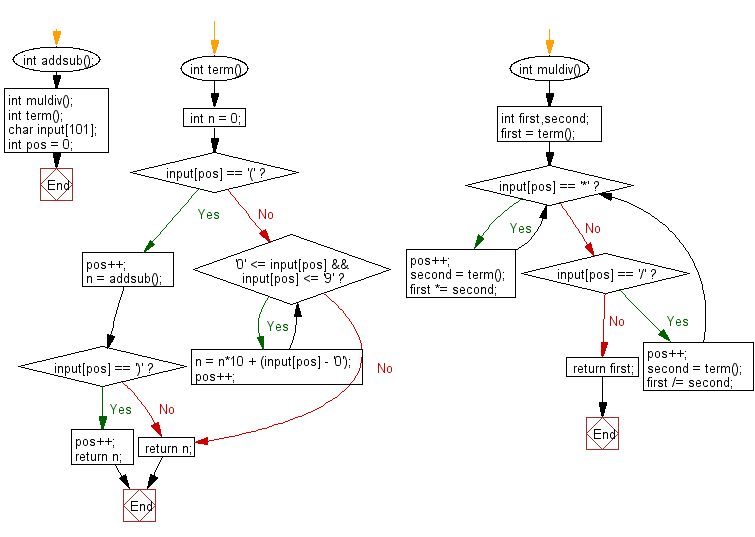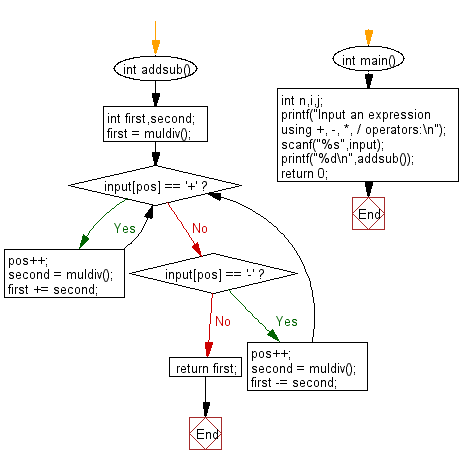﻿ C Program: Reads an expression and evaluates - w3resource

# C Exercises: Reads an expression and evaluates

## C Basic Declarations and Expressions: Exercise-150 with Solution

Write a C program that reads an expression and evaluates it.

Terms and conditions:
The expression consists of numerical values, operators and parentheses, and the ends with '='.
The operators includes +, -, *, / where, represents, addition, subtraction, multiplication and division.
When two operators have the same precedence, they are applied to left to right.
You may assume that there is no division by zero.
All calculation is performed as integers, and after the decimal point should be truncated
Length of the expression will not exceed 100.
-1 × 10 9 <= intermediate results of computation <= 10 9
Sample Input:
4
10-2*3=
8*(8+2-5)=

Sample Solution:

C Code:

``````#include <stdio.h>
#include <string.h>

// Function declarations
int muldiv();
int term();

char input; // Declare character array to store user input
int pos = 0;    // Initialize a global variable to keep track of the position in the input string

// Function to parse and evaluate a term (number or expression inside parentheses)
int term(){
int n = 0;

// Check if the current character is an opening parenthesis
if(input[pos] == '('){
pos++; // Move to the next character
n = addsub(); // Evaluate the expression inside the parentheses

// Check if the next character is a closing parenthesis
if(input[pos] == ')'){
pos++; // Move to the next character (closing parenthesis)
return n; // Return the evaluated result
}
}else{
// If the current character is a digit, parse the number
while('0' <= input[pos] && input[pos] <= '9'){
n = n*10 + (input[pos] - '0'); // Convert characters to integer and build the number
pos++; // Move to the next character
}
}
return n; // Return the parsed number
}

// Function to handle multiplication and division operations
int muldiv(){
int first,second;

first = term(); // Evaluate the first term

for(;;){
if(input[pos] == '*'){
pos++; // Move to the next character (operator)
second = term(); // Evaluate the second term
first *= second; // Perform multiplication
}else if(input[pos] == '/'){
pos++; // Move to the next character (operator)
second = term(); // Evaluate the second term
first /= second; // Perform division
}else{
return first; // If no more operators, return the result
}
}
}

// Function to handle addition and subtraction operations
int first,second;

first = muldiv(); // Evaluate the first term

for(;;){
if(input[pos] == '+'){
pos++; // Move to the next character (operator)
second = muldiv(); // Evaluate the second term
first += second; // Perform addition
}else if(input[pos] == '-'){
pos++; // Move to the next character (operator)
second = muldiv(); // Evaluate the second term
first -= second; // Perform subtraction
}else{
return first; // If no more operators, return the result
}
}
}

int main(){
int n,i,j;
printf("Input an expression using +, -, *, / operators:\n");
scanf("%s",input); // Read the user input as a string
printf("%d\n",addsub()); // Evaluate the expression and print the result
return 0; // Indicate successful execution and return 0 to the operating system
}
``````

Sample Output:

```Input an expression using +, -, *, / operators:
1+6*8-4/2
47
```

Sample Output:

```Input an expression using +, -, *, / operators:
25/5-6*7+2
-35
```

Sample Output:

```Input an expression using +, -, *, / operators:
9+6+(5*2)-5
20
```

Flowchart:C programming Code Editor:

What is the difficulty level of this exercise?

Test your Programming skills with w3resource's quiz.

﻿